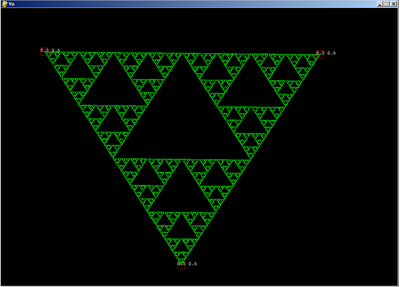# Chaos Game Fractal Generator

Create fractals using the chaos game method.Charlie Harrison
(theratking)

From Wikipedia: In mathematics, the term chaos game, as coined by Michael Barnsley, originally referred to a method of creating a fractal, using a polygon and an initial point selected at random inside it. The fractal is created by iteratively creating a sequence of points, starting with the initial random point, in which each point in the sequence is a given fraction of the distance between the previous point and one of the vertices of the polygon; the vertex is chosen at random in each iteration. Repeating this iterative process a large number of times, selecting the vertex at random on each iteration, and throwing out the first few points in the sequence, will often (but not always) produce a fractal shape. Using a regular triangle and the factor 1/2 will result in the Sierpinski triangle.

Controls:

Q and A control the x-component factor for all vertices

W and S control the y-component factor for all vertices

R and F control the x factor for a highlighted vertex

T and G control the y factor for a highlighted vertex

(EDIT: sorry I totally forgot these essential controls!)

D removes a vertex

If you're looking for inspiration, try making a square with 3 boxes per side and an x and y factor of .7 for Sierpinski's Gasket.

### Changes